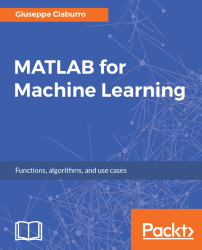•#### MATLAB for Machine Learning#### Overview of this book

MATLAB is the language of choice for many researchers and mathematics experts for machine learning. This book will help you build a foundation in machine learning using MATLAB for beginners. You’ll start by getting your system ready with t he MATLAB environment for machine learning and you’ll see how to easily interact with the Matlab workspace. We’ll then move on to data cleansing, mining and analyzing various data types in machine learning and you’ll see how to display data values on a plot. Next, you’ll get to know about the different types of regression techniques and how to apply them to your data using the MATLAB functions. You’ll understand the basic concepts of neural networks and perform data fitting, pattern recognition, and clustering analysis. Finally, you’ll explore feature selection and extraction techniques for dimensionality reduction for performance improvement. At the end of the book, you will learn to put it all together into real-world cases covering major machine learning algorithms and be comfortable in performing machine learning with MATLAB.
Title PageCreditsForewordwww.PacktPub.comCustomer FeedbackPrefaceFree Chapter
Getting Started with MATLAB Machine LearningImporting and Organizing Data in MATLABFrom Data to Knowledge DiscoveryFinding Relationships between Variables - Regression TechniquesPattern Recognition through Classification AlgorithmsIdentifying Groups of Data Using Clustering MethodsSimulation of Human Thinking - Artificial Neural NetworksImproving the Performance of the Machine Learning Model - Dimensionality ReductionMachine Learning in Practice## Exporting data from MATLAB

Many of the functions we have used to import data into MATLAB have a corresponding function that allows us to export data. At the beginning of the chapter, we learned to save our data for later use with the `save` command:

`>> save filename.mat`

### Note

Remember, this command saves all contents of the workspace in a compressed file with a `.mat` extension, called a MAT-file.

The `dlmread()` function allows us to handle text files with a specified delimiter. We can use this function to write a matrix to an ASCII-delimited file. To test the function, we start from a matrix of random numbers:

```>> MyMatrix = rand(5)
MyMatrix =
0.7577    0.7060    0.8235    0.4387    0.4898
0.7431    0.0318    0.6948    0.3816    0.4456
0.3922    0.2769    0.3171    0.7655    0.6463
0.6555    0.0462    0.9502    0.7952    0.7094
0.1712    0.0971    0.0344    0.1869    0.7547```

Now simply write a matrix named `MyMatrix` to a file named `MyMatrix.txt` using the default delimiter...